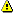# 深度学习利器：TensorFlow实战## TensorFlow变量

TensorFlow中的变量在使用前需要被初始化，在模型训练中或训练完成后可以保存或恢复这些变量值。下面介绍如何创建变量，初始化变量，保存变量，恢复变量以及共享变量。

```  ```#创建模型的权重及偏置

weights = tf.Variable(tf.random_normal([784, 200], stddev=0.35), name="weights")

biases = tf.Variable(tf.zeros(), name="biases")

#指定变量所在设备为CPU:0

with tf.device("/cpu:0"):

v = tf.Variable(...)

#初始化模型变量

init_op = tf.global_variables_initializer()

sess=tf.Session()

sess.run(init_op)

#保存模型变量，由三个文件组成model.data，model.index，model.meta

saver = tf.train.Saver()

saver.restore(sess, "/tmp/model")

#恢复模型变量

saver.restore(sess, "/tmp/model")``````

```  ```#定义卷积神经网络运算规则，其中weights和biases为共享变量

def conv_relu(input, kernel_shape, bias_shape):

# 创建变量"weights".

weights = tf.get_variable("weights", kernel_shape, initializer=tf.random_normal_initializer())

# 创建变量 "biases".

biases = tf.get_variable("biases", bias_shape, initializer=tf.constant_initializer(0.0))

conv = tf.nn.conv2d(input, weights, strides=[1, 1, 1, 1], padding='SAME')

return tf.nn.relu(conv + biases)

#定义卷积层，conv1和conv2为变量命名空间

with tf.variable_scope("conv1"):

# 创建变量 "conv1/weights", "conv1/biases".

relu1 = conv_relu(input_images, [5, 5, 32, 32], )

with tf.variable_scope("conv2"):

# 创建变量 "conv2/weights", "conv2/biases".

relu1 = conv_relu(relu1, [5, 5, 32, 32], )``````

## TensorFlow应用架构

TensorFlow的应用架构主要包括模型构建，模型训练，及模型评估三个方面。模型构建主要指构建深度学习神经网络，模型训练主要指在TensorFlow会话中对训练数据执行神经网络运算，模型评估主要指根据测试数据评估模型精确度。如下图所示：```  ```#两个隐藏层，一个logits输出层

hidden1 = tf.nn.relu(tf.matmul(images, weights) + biases)

hidden2 = tf.nn.relu(tf.matmul(hidden1, weights) + biases)

logits = tf.matmul(hidden2, weights) + biases

#损失方程，采用softmax交叉熵算法

cross_entropy = tf.nn.sparse_softmax_cross_entropy_with_logits( logits, labels, name='xentropy')

loss = tf.reduce_mean(cross_entropy, name='xentropy_mean')

#选定优化算法及定义训练操作

global_step = tf.Variable(0, name='global_step', trainable=False)

train_op = optimizer.minimize(loss, global_step=global_step)

#加载训练数据，并执行网络训练

for step in xrange(FLAGS.max_steps):

feed_dict = fill_feed_dict(data_sets.train, images_placeholder, labels_placeholder)

_, loss_value = sess.run([train_op, loss], feed_dict=feed_dict)

#加载测试数据，计算模型精确度

for step in xrange(steps_per_epoch):

feed_dict = fill_feed_dict(data_set, images_placeholder, labels_placeholder)

true_count += sess.run(eval_correct, feed_dict=feed_dict)``````

## TensorFlow可视化技术TensorFlow可视化技术主要分为两部分：TensorFlow摘要模型及TensorBoard可视化组件。在摘要模型中，需要把模型变量或样本数据转换为TensorFlow summary操作，然后合并summary操作，最后通过Summary Writer操作写入TensorFlow的事件日志。TensorBoard通过读取事件日志，进行相关摘要信息的可视化展示，主要包括：Scalar图、图片数据可视化、声音数据展示、图模型可视化，以及变量数据的直方图和概率分布图。TensorFlow可视化技术的关键流程如下所示：

```  ```#定义变量及训练数据的摘要操作

tf.summary.scalar('max', tf.reduce_max(var))

tf.summary.histogram('histogram', var)

tf.summary.image('input', image_shaped_input, 10)

#定义合并变量操作，一次性生成所有摘要数据

merged = tf.summary.merge_all()

#定义写入摘要数据到事件日志的操作

train_writer = tf.train.SummaryWriter(FLAGS.log_dir + '/train', sess.graph)

test_writer = tf.train.SummaryWriter(FLAGS.log_dir + '/test')

#执行训练操作，并把摘要信息写入到事件日志

summary, _ = sess.run([merged, train_step], feed_dict=feed_dict(True))

#下载示例code，并执行模型训练

python mnist_with_summaries.py

tensorboard --logdir=/path/to/log-directory``````

TensorBoard Scalar图如下所示，其中横坐标表示模型训练的迭代次数，纵坐标表示该标量值，例如模型精确度，熵值等。TensorBoard支持这些统计值的下载。TensorFlow Image摘要信息如下图所示，该示例中显示了测试数据和训练数据中的手写数字图片。TensorFlow图模型如下图所示，可清晰地展示模型的训练流程，其中的每个方框表示变量所在的命名空间。包含的命名空间有input（输入数据），input_reshape（矩阵变换，用于图形化手写数字）, layer1（隐含层1）, layer2（隐含层2）, dropout（丢弃一些神经元，防止过拟合）, accuracy（模型精确度）, cross_entropy（目标函数值，交叉熵）, train（训练模型）。例如，input命名空间操作后的tensor数据会传递给input_reshape，train，accuracy，layer1，cross_entropy命名空间中的操作。TensorFlow变量的概率分布如下图所示，其中横坐标为迭代次数，纵坐标为变量取值范围。图表中的线表示概率百分比，从高到底为[maximum, 93%, 84%, 69%, 50%, 31%, 16%, 7%, minimum]。例如，图表中从高到底的第二条线为93%，对应该迭代下有93%的变量权重值小于该线对应的目标值。## TensorFlow GPU使用

GPU设备已经广泛地应用于图像分类，语音识别，自然语言处理，机器翻译等深度学习领域，并实现了开创性的性能改进。与单纯使用CPU相比，GPU 具有数以千计的计算核心，可实现 10-100 倍的性能提升。TensorFlow支持GPU运算的版本为tensorflow-gpu，并且需要先安装相关软件：GPU运算平台CUDA和用于深度神经网络运算的GPU加速库CuDNN。在TensorFlow中，CPU或GPU的表示方式如下所示：

"/cpu:0"：表示机器中第一个CPU。

"/gpu:0"：表示机器中第一个GPU卡。

"/gpu:1"：表示机器中第二个GPU卡。

TensorFlow中所有操作都有CPU和GPU运算的实现，默认情况下GPU运算的优先级比CPU高。如果TensorFlow操作没有指定在哪个设备上进行运算，默认会优选采用GPU进行运算。下面介绍如何在TensorFlow使用GPU：

```  ```# 定义使用gpu0执行a*b的矩阵运算，其中a，b，c都在gpu0上执行

with tf.device('/gpu:0'):

a = tf.constant([1.0, 2.0, 3.0, 4.0, 5.0, 6.0], shape=[2, 3], name='a')

b = tf.constant([1.0, 2.0, 3.0, 4.0, 5.0, 6.0], shape=[3, 2], name='b')

c = tf.matmul(a, b)

# 通过log_device_placement指定在日志中输出变量和操作所在的设备

sess = tf.Session(config=tf.ConfigProto(log_device_placement=True))

print sess.run(c)``````

```  ```#设备的Device Mapping

/job:localhost/replica:0/task:0/gpu:0 -> device: 0, name: Tesla K20c, pci bus id: 0000:81:00.0

#变量操作所在设备位置

```  ```#定义TensorFlow配置

config = tf.ConfigProto()

#配置GPU内存分配方式

#config.gpu_options.allow_growth = True

#config.gpu_options.per_process_gpu_memory_fraction = 0.4

session = tf.Session(config=config, ...)``````

## TensorFlow与HDFS集成使用

HDFS是一个高度容错性的分布式系统，能提供高吞吐量的数据访问，非常适合大规模数据集上的应用。TensorFlow与HDFS集成示例如下：

```  ```#配置JAVA和HADOOP环境变量

export LD_LIBRARY_PATH=\$LD_LIBRARY_PATH:\$JAVA_HOME/jre/lib/amd64/server

#执行TensorFlow运行模型

#在TensorFlow模型中定义文件的读取队列

filename_queue = tf.train.string_input_producer(["hdfs://namenode:8020/path/to/file1.csv", "hdfs://namenode:8020/path/to/file2.csv"])

#从文件中读取一行数据，value为所对应的行数据

# 把读取到的value值解码成特征向量，record_defaults定义解码格式及对应的数据类型

record_defaults = [, , , , ]

col1, col2, col3, col4, col5 = tf.decode_csv(value, record_defaults=record_defaults)

features = tf.pack([col1, col2, col3, col4])

with tf.Session() as sess:

# 定义同步对象，并启动相应线程把HDFS文件名插入到队列

coord = tf.train.Coordinator()

for i in range(1200):

# 从文件队列中读取一行数据

example, label = sess.run([features, col5])

#请求停止队列的相关线程（包括进队及出队线程）

coord.request_stop()

#等待队列中相关线程结束（包括进队及出队线程）